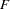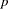The CANCORR Procedure

# Getting Started: CANCORR Procedure

The following example demonstrates how you can use the CANCORR procedure to calculate and test canonical correlations between two sets of variables.

Suppose you want to determine the degree of correspondence between a set of job characteristics and measures of employee satisfaction. Using a survey instrument for employees, you calculate three measures of job satisfaction. With another instrument designed for supervisors, you calculate the corresponding job characteristics profile.

Your three variables associated with job satisfaction are as follows:

• career track satisfaction: employee satisfaction with career direction and the possibility of future advancement, expressed as a percent

• management and supervisor satisfaction: employee satisfaction with supervisor’s communication and management style, expressed as a percent

• financial satisfaction: employee satisfaction with salary and other benefits, using a scale measurement from 1 to 10 (1=unsatisfied, 10=satisfied)

The three variables associated with job characteristics are as follows:

• task variety: degree of variety involved in tasks, expressed as a percent

• feedback: degree of feedback required in job tasks, expressed as a percent

• autonomy: degree of autonomy required in job tasks, expressed as a percent

The following statements create the SAS data set Jobs and request a canonical correlation analysis:

```   data Jobs;
input Career Supervisor Finance Variety Feedback Autonomy;
label Career    ='Career Satisfaction' Variety ='Task Variety'
Supervisor='Supervisor Satisfaction' Feedback='Amount of Feedback'
Finance   ='Financial Satisfaction' Autonomy='Degree of Autonomy';
datalines;
72  26  9          10  11  70
63  76  7          85  22  93
96  31  7          83  63  73
96  98  6          82  75  97
84  94  6          36  77  97
66  10  5          28  24  75
31  40  9          64  23  75
45  14  2          19  15  50
42  18  6          33  13  70
79  74  4          23  14  90
39  12  2          37  13  70
54  35  3          23  74  53
60  75  5          45  58  83
63  45  5          22  67  53
;
```
```    proc cancorr data=Jobs
vprefix=Satisfaction wprefix=Characteristics
vname='Satisfaction Areas' wname='Job Characteristics';
var  Career Supervisor Finance;
with Variety Feedback Autonomy;
run;

```

The DATA= option in the PROC CANCORR statement specifies Jobs as the SAS data set to be analyzed. The VPREFIX and WPREFIX options specify the prefixes for naming the canonical variables from the VAR statement and the WITH statement, respectively. The VNAME option specifies ‘Satisfaction Areas’ to refer to the set of variables from the VAR statement. Similarly, the WNAME option specifies ‘Job Characteristics’ to refer to the set of variables from the WITH statement.

The VAR statement defines the first of the two sets of variables to be analyzed as Career, Supervisor, and Finance. The WITH statement defines the second set of variables to be Variety, Feedback, and Autonomy. The results of this analysis are displayed in Figure 26.1 to Figure 26.4.

Figure 26.1 displays the canonical correlation, adjusted canonical correlation, approximate standard error, and squared canonical correlation for each pair of canonical variables. The first canonical correlation (the correlation between the first pair of canonical variables) is 0.9194. This value represents the highest possible correlation between any linear combination of the job satisfaction variables and any linear combination of the job characteristics variables.

Figure 26.1 also lists the likelihood ratio and associated statistics for testing the hypothesis that the canonical correlations in the current row and all that follow are zero.

Figure 26.1 Canonical Correlations, Eigenvalues, and Likelihood Tests
The CANCORR Procedure

Canonical Correlation Analysis

Canonical
Correlation
Canonical
Correlation
Approximate
Standard
Error
Squared
Canonical
Correlation
Eigenvalues of Inv(E)*H
= CanRsq/(1-CanRsq)
Test of H0: The canonical correlations in the current row and all that follow are zero
Eigenvalue Difference Proportion Cumulative Likelihood
Ratio
Approximate
F Value
Num DF Den DF Pr > F
1 0.919412 0.898444 0.042901 0.845318 5.4649 5.2524 0.9604 0.9604 0.12593148 2.93 9 19.621 0.0223
2 0.418649 0.276633 0.228740 0.175267 0.2125 0.1995 0.0373 0.9977 0.81413359 0.49 4 18 0.7450
3 0.113366 . 0.273786 0.012852 0.0130   0.0023 1.0000 0.98714819 0.13 1 10 0.7257

The first approximatevalue of 2.93 corresponds to the test that all three canonical correlations are zero. Since the-value is small (0.0223), you would reject the null hypothesis at the 0.05 level. The second approximatevalue of 0.49 corresponds to the test that both the second and the third canonical correlations are zero. Since the-value is large (0.7450), you would fail to reject the hypothesis and conclude that only the first canonical correlation is significant.

Figure 26.2 lists several multivariate statistics and tests that use approximations based on thedistribution for the null hypothesis that all canonical correlations are zero. Alternatively, you can specify MSTAT=EXACT to compute exact-values for three of the four tests (Wilks’ Lambda, the Hotelling-Lawley Trace, and Roy’s greatest root) and an improvedapproximation for the fourth (Pillai’s Trace). These statistics are described in the section Multivariate Tests in Chapter 4, Introduction to Regression Procedures.

Figure 26.2 Multivariate Statistics andApproximations
Multivariate Statistics and F Approximations
S=3 M=-0.5 N=3
Statistic Value F Value Num DF Den DF Pr > F
Wilks' Lambda 0.12593148 2.93 9 19.621 0.0223
Pillai's Trace 1.03343732 1.75 9 30 0.1204
Hotelling-Lawley Trace 5.69042615 4.76 9 9.8113 0.0119
Roy's Greatest Root 5.46489324 18.22 3 10 0.0002

The small-values for these tests (< 0.05), except for Pillai’s trace, suggest rejecting the null hypothesis that all canonical correlations are zero in the population, confirming the results of the preceding likelihood ratio test (Figure 26.1). With only one of the tests resulting in a-value larger than 0.05, you can assume that the first canonical correlation is significant. The next step is to interpret or identify the two canonical variables corresponding to this significant correlation.

Even though canonical variables are artificial, they can often be "identified" in terms of the original variables. This is done primarily by inspecting the standardized coefficients of the canonical variables and the correlations between the canonical variables and their original variables. Since only the first canonical correlation is significant, only the first pair of canonical variables (Satisfaction1 and Characteristics1) need to be identified.

PROC CANCORR calculates and displays the raw canonical coefficients for the job satisfaction variables and the job characteristic variables. However, since the original variables do not necessarily have equal variance and are not measured in the same units, the raw coefficients must be standardized to allow interpretation. The coefficients are standardized by multiplying the raw coefficients with the standard deviation of the associated variable.

The standardized canonical coefficients in Figure 26.3 show that the first canonical variable for the Satisfaction group is a weighted sum of the variables Supervisor (0.7854) and Career (0.3028), with the emphasis on Supervisor. The coefficient for the variable Finance is near 0. Thus, a person satisfied with his or her supervisor and with a large degree of career satisfaction would score high on the canonical variable Satisfaction1.

Figure 26.3 Standardized Canonical Coefficients from the CANCORR Procedure
Standardized Canonical Coefficients for the Satisfaction Areas
Satisfaction1 Satisfaction2 Satisfaction3
Career Career Satisfaction 0.3028 -0.5416 1.0408
Supervisor Supervisor Satisfaction 0.7854 0.1305 -0.9085
Finance Financial Satisfaction 0.0538 0.9754 0.3329

Standardized Canonical Coefficients for the Job Characteristics
Characteristics1 Characteristics2 Characteristics3
Variety Task Variety -0.1108 0.8095 0.9071
Feedback Amount of Feedback 0.5520 -0.7722 0.4194
Autonomy Degree of Autonomy 0.8403 0.1020 -0.8297

The coefficients for the job characteristics variables show that degree of autonomy (Autonomy) and amount of feedback (Feedback) contribute heavily to the Characteristics1 canonical variable (0.8403 and 0.5520, respectively).

Figure 26.4 shows the table of correlations between the canonical variables and the original variables.

Figure 26.4 Canonical Structure Correlations from the CANCORR Procedure
The CANCORR Procedure

Canonical Structure

Correlations Between the Satisfaction Areas and Their Canonical Variables
Satisfaction1 Satisfaction2 Satisfaction3
Career Career Satisfaction 0.7499 -0.2503 0.6123
Supervisor Supervisor Satisfaction 0.9644 0.0362 -0.2618
Finance Financial Satisfaction 0.2873 0.8814 0.3750

Correlations Between the Job Characteristics and Their Canonical Variables
Characteristics1 Characteristics2 Characteristics3
Variety Task Variety 0.4863 0.6592 0.5736
Feedback Amount of Feedback 0.6216 -0.5452 0.5625
Autonomy Degree of Autonomy 0.8459 0.4451 -0.2938

Correlations Between the Satisfaction Areas and the Canonical Variables of the Job Characteristics
Characteristics1 Characteristics2 Characteristics3
Career Career Satisfaction 0.6895 -0.1048 0.0694
Supervisor Supervisor Satisfaction 0.8867 0.0152 -0.0297
Finance Financial Satisfaction 0.2642 0.3690 0.0425

Correlations Between the Job Characteristics and the Canonical Variables of the Satisfaction Areas
Satisfaction1 Satisfaction2 Satisfaction3
Variety Task Variety 0.4471 0.2760 0.0650
Feedback Amount of Feedback 0.5715 -0.2283 0.0638
Autonomy Degree of Autonomy 0.7777 0.1863 -0.0333

Although these univariate correlations must be interpreted with caution since they do not indicate how the original variables contribute jointly to the canonical analysis, they are often useful in the identification of the canonical variables.

Figure 26.4 shows that the supervisor satisfaction variable Supervisor is strongly associated with the Satisfaction1 canonical variable, with a correlation of 0.9644. Slightly less influential is the variable Career, which has a correlation with the canonical variable of 0.7499. Thus, the canonical variable Satisfaction1 seems to represent satisfaction with supervisor and career track.

The correlations for the job characteristics variables show that the canonical variable Characteristics1 seems to represent all three measured variables, with degree of autonomy variable (Autonomy) being the most influential (0.8459).

Hence, you can interpret these results to mean that job characteristics and job satisfaction are related—jobs that possess a high degree of autonomy and level of feedback are associated with workers who are more satisfied with their supervisor and their career. While financial satisfaction is a factor in job satisfaction, it is not as important as the other measured satisfaction-related variables.Previous Page | Next Page | Top of Page